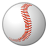# QlikView App Dev

Discussion Board for collaboration related to QlikView App Development.

Announcements
Welcome to Qlik Community! Check out our new navigation! FIND OUT MORE
cancel
Showing results for
Did you mean:Contributor III

## Replace or change value in straight table

Hi everybody,

In a straight table, I'd like to replace the value of a field when the value is 0.

Here is the expression that I created :

=if(\$(v_Qty)=0,(\$(v_Total_Qty)/\$(v_NbSalesmen),\$(v_Qty))

This is what I get in my straight table :

 Material Code SalesRepCode Qty Total Qty if(\$(v_Qty)=0,(\$(v_Qty_Total)/\$(v_NbSalesmen)),\$(v_Qty)) 270 270 270 1 PO 52 148 52 1 PR 96 148 96 1 PT 0 148 49,33 2 PO 33 122 33 2 PR 75 122 75 2 PT 14 122 14

The problem is that the substitute value ( here it's 49.33) is not taken into account in the total of the new expression. Instead of 270, I should have 319,33. I know that I can choose the option "Sum of rows". With it, I do have the good result.

My problem is that I need to calculate a new Total quantity with this expression, so this solution does not solve my problem.

I also tried : if(\$(v_Qty)=0,\$(v_Qty)+(\$(v_Qty_Total)/\$(v_NbSalesmen)),\$(v_Qty)) but the result is the same

For my new Total Qty, the forum helped me find an expression that works

Sum(TOTAL<Material_Code>Aggr(if(\$(v_Qty_M3_LY)=0,(\$(v_Qty_Totalparproduit_LY)/\$(v_Nb_ToutVendeurs)),\$(v_Qty_M3_LY)),[Material_Code],[SalesRep_Code]))

But I need to fix the result of this, whatever the Sales Representant I choose to filter.

You can check the result that I'm looking for in the attached excel file.

Loreen

8 RepliesMVP

So, are you looking to get 'New total qty' column from the Excel file and this didn't do it?

`Sum(TOTAL<Material_Code>Aggr(if(\$(v_Qty_M3_LY)=0,(\$(v_Qty_Totalparproduit_LY)/\$(v_Nb_ToutVendeurs)),\$(v_Qty_M3_LY)),[Material_Code],[SalesRep_Code]))`Specialist

Hi Loreen.

Expressions for:

Total_Qty = Sum(distinct Aggr(nodistinct Sum([Qty]),[Product n°]))

Total_Qty1 = Sum(if(Qty=0,(Aggr(nodistinct Sum([Qty]),[Product n°]))/3,Qty))

New_Total = Sum(distinct Aggr(nodistinct Sum(if(Qty=0,(Aggr(nodistinct Sum([Qty]),[Product n°]))/3,Qty)),[Product n°]))Contributor III
Author

Yes that expression works. But i'd like that  results do not change when I filter the Sales Representant.

I'd like to have this :

 Product n° Salesman Qty Total Qty if(\$(v_Qty)=0,(\$(v_Qty_Total)/\$(v_NbSalesmen)),\$(v_Qty_M3C)) New total qty 1 PO 52 148 52 197,3333333 2 PO 33 122 33 122

But instead I have

 Product n° Salesman Qty Total Qty if(\$(v_Qty)=0,(\$(v_Qty_Total)/\$(v_NbSalesmen)),\$(v_Qty_M3C)) New total qty 1 PO 52 148 52 52 2 PO 33 122 33 33Contributor III
Author

Thanks for your reply. My Qty field is an expression, not a dimension so I can't make a sum with it.MVP

How do you define these variables

\$(v_Qty_M3_LY)

\$(v_Qty_Totalparproduit_LY)

\$(v_Nb_ToutVendeurs)Specialist

But you have a field with this data right? so you can work with it, even in that table it is an expression.  I just took your data from the excel file.  Anyway you can take the expression I sent to you and modify them.Contributor III
Author

My analysis focuses on 17 salesmen ( salesmen = vendeurs)

\$(v_Nb_Toutvendeurs) = 17

I created an expression that gives me the quantity sold but only those 17 salesmen : \$(v_Qty_17vendeurs)

\$(v_Qty_M3C_Y_LY) = this is the quantity of the last year

\$(v_Qty_Totalparproduit_LY) = Sum(Aggr(NODISTINCT(\$(v_Qty_17vendeurs_Y_LY)),Material_Code))

\$(v_Qty_M3_LY) = if(\$(v_Qty_M3C_Y_LY)=0,(\$(v_Qty_Totalparproduit_LY)/\$(v_Nb_Toutvendeurs)),\$(v_Qty_M3C_Y_LY))Specialist
Its difficult to give you a solution with this variables in the middle of expression. I suggest that you try to put your code directly inside the expression in the table and then convert back to variable.

In addition you want to that the result remain as you select some filters. in this case it is necessary to use set analysis.